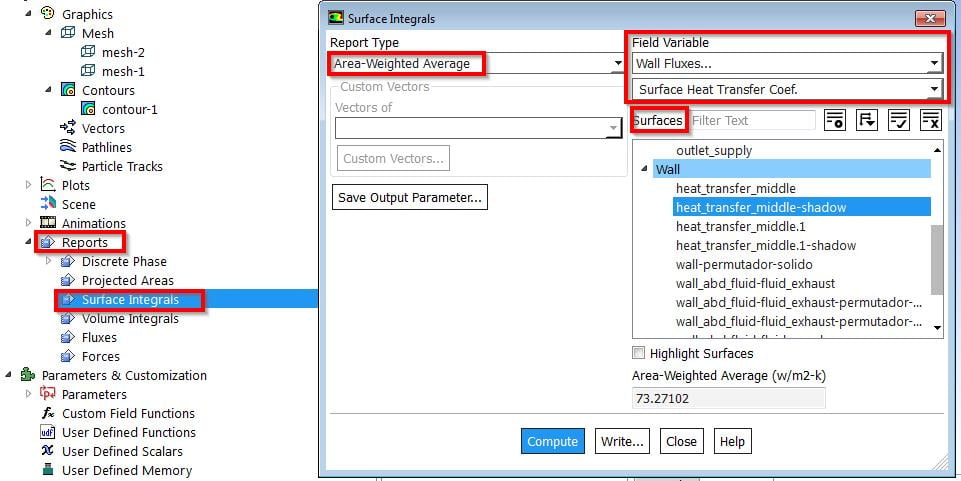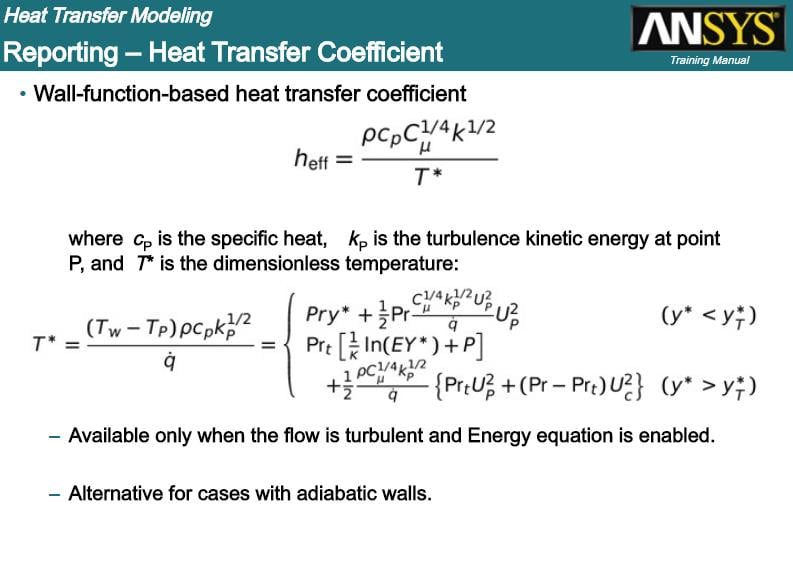## Fluids

•hugo CFD
Subscriber

Hello.

I have some doubts that i would like to clarify. Consider a fixed plate heat exchanger air-air with quasi counter flow. The heat exchange is through forced convection.

How should I calculate the coefficient of heat transfer? Directly from the fluent across the surfaces integrals --> area weighted average or through the formula h = q / at, where do I take the values of twall and tref and q from the fluent?

The next question is about boundary conditions. when defining the boundary conditions, is it necessary to consider heat transfer by convection between the air and the plate, by selecting the CONVECTION point in the THERMAL conditions?

Thanks

•Raef.Kobeissi
Subscriber

Hello Hugo,

Question 2 first: It depends on how you're simulating your case, if it is forced convection I would assume air flow is involved around the fixed plate HE and in this case you don't need to use convection.

Question1: I am assuming that you are using a solid wall(meshed) to simulate the HE, in that case you can calculate it through fluent as you mentioned. Can you attach the case file and I can show you how to do it through a small video or series of pictures.

Regards

•hugo CFD
Subscriber

Hello

I am sending the study case. I have read that in certain situations, a UDF is required to calculate h. Am i correct? What are these situations?

Regards

•raul.raghav
Subscriber

For laminar flow in Ansys Fluent, the convective heat transfer coefficient (h) is calculated based on the equation:

h = q / (T_wall - T_ref); where T_ref is the reference temperature you provide. You need to be careful providing the T_ref value, as that can result in the underestimation or overestimation of convective heat transfer coefficient (h) value. I'd highly recommend you to go through the following paper which compares the convective heat transfer coefficient obtained with T_ref value evaluated in different ways:

Determination of surface convective heat transfer coefficients by CFD

You can plot contours of the local heat transfer coefficients. And you can use "Surface integrals" to plot the average heat transfer coefficient (see image below):PS: I haven't checked any of the inputs or outputs in your case file, so I won't be commenting on the results.

•Raef.Kobeissi
Subscriber

Hi Hugo, have you been able to resolve your issue?

•hugo CFD
Subscriber

No. I am looking for another way to get heat transfer coefficient, instead to calculate by T ref method.

Hugo

•raul.raghav
Subscriber

Hugo, as far as I'm aware of, heat transfer coefficient can either be calculated using the standard definition as I mentioned my previous post or by the Reynolds Analogy as turbulent heat transfer is modeled using the analogy.

From the Reynolds analogy: h = (Cf/2) * density * U_ref * Cp

where Cf is the skin friction coefficient, and U_ref is the reference velocity, which is difficult to be estimated. For turbulent flows, you can use wall functions to evaluate h:•hugo CFD
Subscriber

yes. you are right. maybe i didn´t express well.

i considered follow expression to calculte heat transfer coefficient: h = q / ( T _ wall - T _ adj)

q is heat flux and I get this value from fluent (surface integrals---wall flux--- total surface heat flux) referring to the boundary condition "heat transfer 1".

T_wall is a temperature of the wall and i get this value from fluent referring to the boundary condition ""heat transfer 1-shadow".

T_adj is the temperature of the first fluid layer following the wall referring to the boundary condition "heat transfer 1".

My problem is how i get the values of the expression from fluent. I don t know if what i have considered is right.

Hugo

•raul.raghav
Subscriber
•hugo CFD
Subscriber
•raul.raghav
Subscriber

The heat transfer coefficient that is area-weighted average is the average heat transfer coefficient. The contour plot shows the local heat transfer coefficient. And the heat transfer coefficient depends on the fluid, hydrodynamic and thermal conditions and also on the geometry under consideration.

I'm not sure why the coefficient is negative though. Again as I mentioned in my earlier posts, the way you calculate the coefficient makes a whole lot of difference.

•hugo CFD
Subscriber

Hi.

How can I obtain/calculate the local heat transfer coefficient along the length of the heat exchanger? And make a chart of this behavior?

hugo

•raul.raghav
Subscriber
•atulsingh92
Subscriber

@Raul.raghav Could you please explain in more detail, as to how, can one define this reference temperature? The thing is, this value, is good enough to make the nusselt number negative.

So, lets say I am simulating for internal flow in a corrugated tube with periodic bc. (yes i have segregated solver and const heat flux bc as mentioned in Ansys manual). The reference temperature I have chosen, is by reverse calculating the nusselt number mentioned in an experimental paper. i.e the paper mentiones the fluid -water entering at 26 degrees C and then showed a nusselt of 65 for example. The q applied is 21100.

This would leave me by htc= 21100 / (26 - x); Nu = 60 = htc * 0.00575 / 0.61; I enter this x as my reference temperature.

But the question is , here, I knew my nusselt from an experimental data, what to give when I dont know this before hand
Also, the way I mentioned, is it the correct way?

•irineupetri
Subscriber

Hi.

Follow the advice from colleagues above, but don't forget to consider the outer walls of your adiabatic system, setting the heat flux equal to zero (Q = 0) on the external walls.

Good luck

•Mughees Shahid
Subscriber

how we can use convection heat transfer coefficient value in cfd ansys workbench 19 need guidance Thank you

•Maisha Nusrat
Subscriber

Hello I have been facing a problem regarding heat transfer coefficient. I installed 2023 R1 version yesterday. Then I run the simulation using water and a nanofluid. After the simulation is over, I tried to determine area average of surface of heat transfer coefficient on wall. But it keeps showing me error repeatedly and isnt giving me any sort of value. But all these days I was using an older version of ansys where none of these errors were shown.

I really need help right now. Even in data qualities, I have chosen surface heat transfer coefficient saveral times but in the results, it is not showing this parameter.

Can someone help?

Is there other way to determine the coefficient?